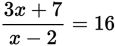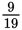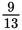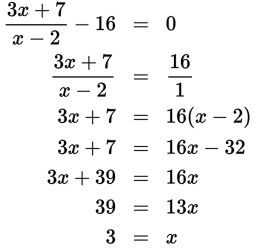# SAT Math Multiple Choice Question 454: Answer and Explanation

### Test Information

Question: 454

4.Which value of x satisfies the equation given above?

• A.• B.• C. 2
• D. 3

Explanation:

D

Difficulty: Easy

Category: Passport to Advanced Math / Exponents

Strategic Advice: Don't let this rational equation intimidate you. Rather than finding a common denominator, move the 16 to the other side of the equation, write it over 1, and use cross-multiplication. Don't forget to check that your solution doesn't cause the denominator to be 0. If it does, then it's an invalid solution.

Getting to the Answer: After moving the 16, cross-multiply and then solve for x using inverse operations.When substituted into the denominator of the original equation, 3 does not cause division by 0, so it is a valid solution.